# Orbital trajectory problem

so I’m trying to make a trajectory for orbiting object but the output(blue dot) is like this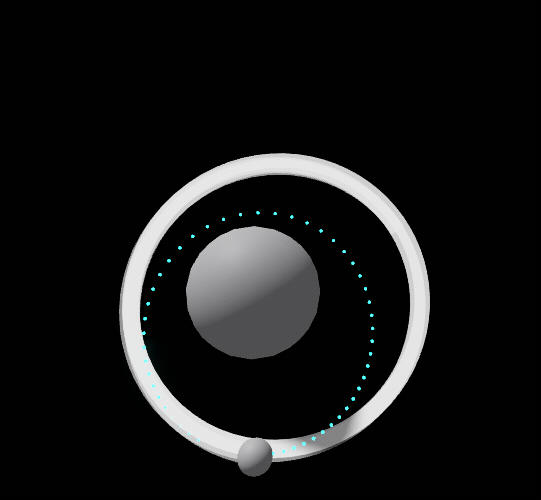note: I give the object initial velocity(assemblylinearvelocity) so it orbit. the white path is from trails, I’m using the trail to see how the trajectory path should be.

what I want is the trajectory is the same as the white path. also, I want to make sure that the blue dot is enough for any type of orbit so the orbit trajectory not like this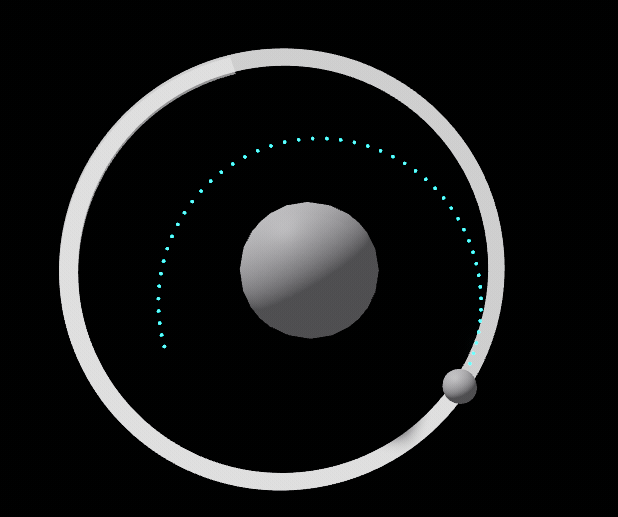my setup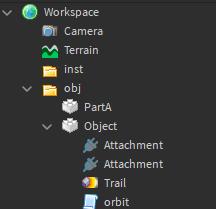here is the script (child of the object)

``````--PartA is anchored
while wait(0.05) do
--gravity effect
local arah = (script.Parent.Position - script.Parent.Parent.PartA.Position).Unit --direction
local force = 10 * script.Parent.Parent.PartA:GetMass() * script.Parent:GetMass() / (script.Parent.Position - script.Parent.Parent.PartA.Position).Magnitude ^ 2 -- Im using newtonial gravity equation, 10 is the constant
script.Parent.AssemblyLinearVelocity -= arah * force * 0.05/script.Parent:GetMass()

--trajectory stuff
fakeVel = script.Parent.AssemblyLinearVelocity
fakePos = script.Parent.Position
workspace.inst:ClearAllChildren() --clear the old blue dot trajectory
for i = 0,50,1 do
local dis = (fakePos - script.Parent.Parent.PartA.Position).Unit
local force = 10 * script.Parent.Parent.PartA:GetMass() * script.Parent:GetMass() / (fakePos - script.Parent.Parent.PartA.Position).Magnitude ^ 2
fakeVel -= dis * force * 0.05/script.Parent:GetMass() -- 0.05 is the delta time
fakePos = fakePos + fakeVel * 0.05 -- 0.05 is the delta time
local a = Instance.new("Part",workspace.inst)
a.Position = fakePos
a.Size = Vector3.new(0.1,0.1,0.1)
a.CanCollide = false
a.Anchored = true
a.Material = "Neon"
a.Color = Color3.fromRGB(87, 255, 250)
a.Shape = "Ball"
end
end

``````

1 Like

The math looks right - I think your problem is that you are assuming that there is .05 time between each “step” of the orbiting object.

``````while true do
wait(.05)
local time1 = tick()
--do logic that takes x amount of time to actually compute & run on the server
local time2 = tick()
end
``````

Total time will actually be .05 + (time2 - time1)
So your blue dots are estimating a path that is actually “faster” than the white orbiting object, and therefore the blue orbit path is closer to the center object.
I would do something like this instead…
Reference: RunService.Stepped

``````local RunService = game:GetService("RunService")

local RATE_PER_SECOND = .05

RunService.Stepped:Connect(function(time, step)
local increment = RATE_PER_SECOND * step
end)``````

Saw immediately what your problem was, you are using euler integration. Gotta update the velocity first then use that to update the position (dont use body movers) also fakeVel is +=

the orbit looks more accurate with this but the blue dot is so short even all of them inside the orbiting object

``````local RunService = game:GetService("RunService")
local Rate_Per_Second = 0.05

RunService.Stepped:Connect(function(times,step)
local increment = Rate_Per_Second * step

local arah = (script.Parent.Position - script.Parent.Parent.PartA.Position).Unit --distance
local force = 20 *script.Parent.Parent.PartA:GetMass() * script.Parent:GetMass() / (script.Parent.Position - script.Parent.Parent.PartA.Position).Magnitude ^ 2
script.Parent.AssemblyLinearVelocity -= arah * force * increment/script.Parent:GetMass()

--trajectory stuff
local fakeVel = script.Parent.AssemblyLinearVelocity
local fakePos = script.Parent.Position
workspace.inst:ClearAllChildren()
for i = 0,50,1 do
local dis = (fakePos - script.Parent.Parent.PartA.Position).Unit
local force = 20 *script.Parent.Parent.PartA:GetMass() * script.Parent:GetMass() / (fakePos - script.Parent.Parent.PartA.Position).Magnitude ^ 2
fakeVel -= dis * force * (increment)/script.Parent:GetMass()
fakePos = fakePos + fakeVel * (increment)
local a = Instance.new("Part",workspace.inst)
a.Position = fakePos
a.Size = Vector3.new(0.1,0.1,0.1)
a.CanCollide = false
a.Anchored = true
a.Material = "Neon"
a.Color = Color3.fromRGB(87, 255, 250)
a.Shape = "Ball"
end
end)
``````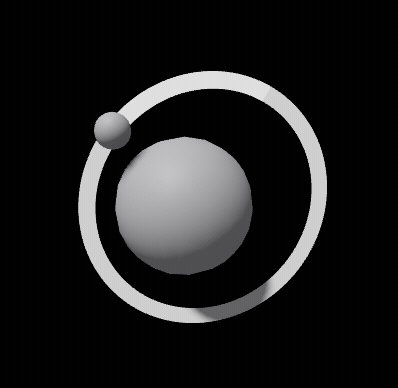and if I increase the for loop to 300 the output is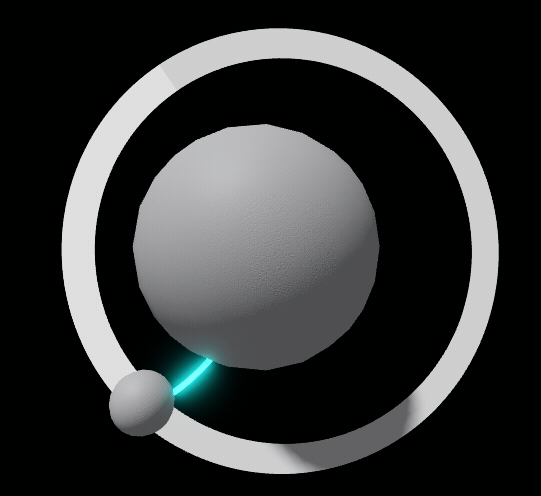I didn’t know about Euler integrationI’m still in high school. I don’t get what you mean about updating velocity first I think I have already done it on my script. if the fakeVel += the output look like thisthis is my script

``````while wait(0.05) do
--gravity effect
time1 = tick()
local arah = (script.Parent.Position - script.Parent.Parent.PartA.Position).Unit --distance
local force = 10 * script.Parent.Parent.PartA:GetMass() * script.Parent:GetMass() / (script.Parent.Position - script.Parent.Parent.PartA.Position).Magnitude ^ 2
script.Parent.AssemblyLinearVelocity -= arah * force * 0.05/script.Parent:GetMass()
time2 = tick()
--trajectory stuff
fakeVel = script.Parent.AssemblyLinearVelocity
fakePos = script.Parent.Position
workspace.inst:ClearAllChildren()
for i = 0,50,1 do
local dis = (fakePos - script.Parent.Parent.PartA.Position).Unit
local force = 10 * script.Parent.Parent.PartA:GetMass() * script.Parent:GetMass() / (fakePos - script.Parent.Parent.PartA.Position).Magnitude ^ 2
fakeVel += dis * force * (0.05 + (time2-time1))/script.Parent:GetMass()
fakePos = fakePos + fakeVel * (0.05 + (time2-time1))
local a = Instance.new("Part",workspace.inst)
a.Position = fakePos
a.Size = Vector3.new(0.1,0.1,0.1)
a.CanCollide = false
a.Anchored = true
a.Material = "Neon"
a.Color = Color3.fromRGB(87, 255, 250)
a.Shape = "Ball"
end
end
``````

I’ve made a few orbital physics simulations I can link you all of them so you can “analyze” my code in a sense

Used WeTransfer cause devforum washttps://we.tl/t-znZrgkDAk5

1 Like

I was able to do this using kepler motion, rather than calculating force/acceleration/velocity/position for each frame. Like in the video @CoderHusk posted, there will always be some noise when you process motion that way. This script can be used to determine orbital parameters beforehand, then calculate true anomaly + distance as a function of time. There will be no noise affecting the path that way.

https://www.roblox.com/library/4829401641/Orbit-Kit

Example place where this script is used:

https://www.roblox.com/games/4888188585/Newtonia

1 Like